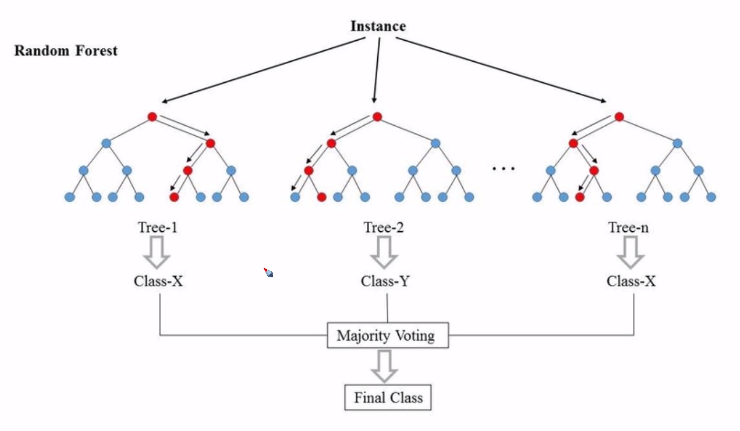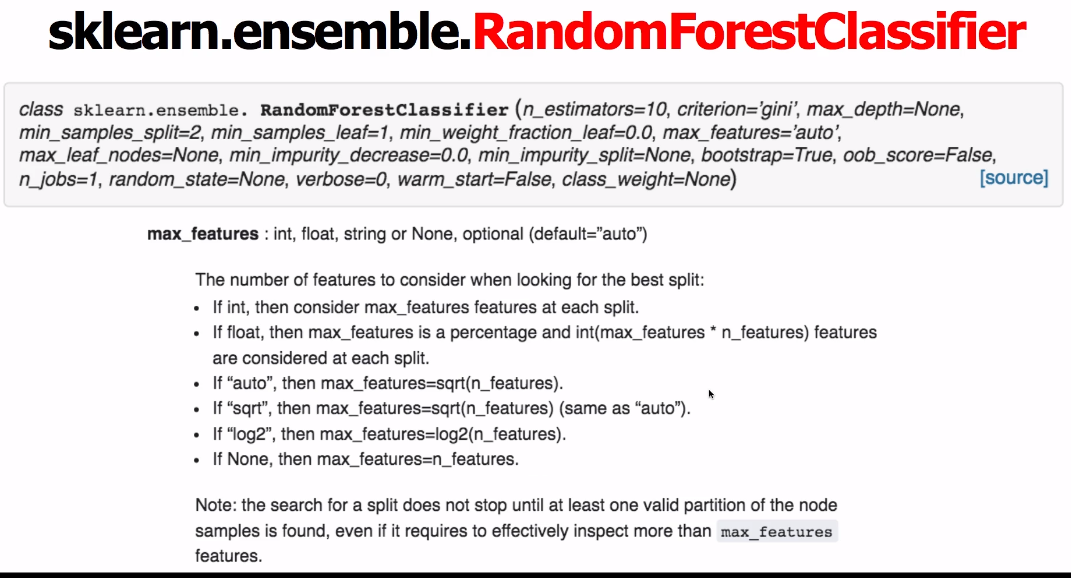# Overview Random Forest

2018, Oct 03

### Random Forest = Bagging + Randomized Decision Tree

• Ensemble of high variance decision trees
• Forest term comes from Combination of trees
• Random Forest is simple but has good performance
• Random Forest supports Regressor and Classifier

In analysis, we can use Random Forest instead of naive Decision Tree.As above, Random Forest consists of many trees which have different shape. In order to make better performance, the shape of each tree should be different. Each tree predicts classification or regression and the Random Forest make result with majority voting.

Random Forest has characteristics

• Learning with m subset data which has low correlation.
• Trees are binary
• Samples n random features to make tree
• If the number of features is p and the number of selected features in the tree is closed to p (n = p), It would close to bagging tree.
• Reuses selected features and common ratio of p is p = sqrt(n) in classifier and p = n/3 in regressor.
• High variance tree is better. The number of last node is between 1 and 5. (In sklearn)

High variance model results in overfitting. But in ensemble, Random Forest, It’s the beauty of importance. Each overfitting result of trees comes together and In the perspective of whole, It works out. Accordingly, In random forest, we are to make deep depth tree.from sklearn.ensemble import RandomForestClassifier
eclf_rf = RandomForestClassifier(n_estimators=100)
cross_val_score(eclf, X, y, cv = 10).mean()